### 13.2.1.3 Higher order differential constraints

The phase space idea can even be applied to differential equations with order higher than two. For example, a constraint may involve the time derivative of acceleration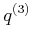, which is often called jerk. If the differential equations involve jerk variables, then a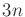-dimensional phase space can be defined to obtain first-order constraints. In this case, each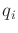,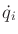, and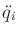in a constraint such as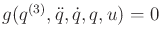is defined as a phase variable. Similarly,th-order differential constraints can be reduced to first-order constraints by introducing a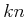-dimensional phase space.

Example 13..4 (Chain of Integrators)   A simple example of higher order differential constraints is the chain of integrators.13.6 This is a higher order generalization of Example 13.3. Suppose that ath-order differential equation is given as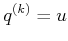, in which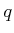andare scalars, and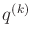denotes theth derivative ofwith respect to time.

A-dimensional phase spaceis defined in which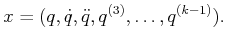(13.36)

The state (or phase) transition equation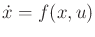is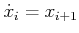for eachsuch that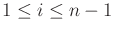, and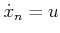. Together, theseindividual equations are equivalent to.

The initial state specifies the initial position and all time derivatives up to order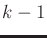. Using these and the action, the state trajectory can be obtained by a chain of integrations.You might be wondering whether derivatives can be eliminated completely by introducing a phase space that has high enough dimension. This does actually work. For example, if there are second-order constraints, then a-dimensional phase space can be introduced in which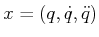. This enables constraints such as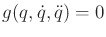to appear as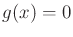. The trouble with using such formulations is that the state must follow the constraint surface in a way that is similar to traversing the solution set of a closed kinematic chain, as considered in Section 4.4. This is why tangent spaces arose in that context. In either case, the set of allowable velocities becomes constrained at every point in the space.

Problems defined using phase spaces typically have an interesting property known as drift. This means that for some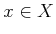, there does not exist any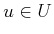such that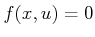. For the examples in Section 13.1.2, such an action always existed. These were examples of driftless systems. This was possible because the constraints did not involve dynamics. In a dynamical system, it is impossible to instantaneously stop due to momentum, which is a form of drift. For example, a car will drift'' into a brick wall if it is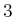meters way and traveling 100 km/hr in the direction of the wall. There exists no action (e.g., stepping firmly on the brakes) that could instantaneously stop the car. In general, there is no way to instantaneously stop in the phase space.

Steven M LaValle 2020-08-14